# RS Aggarwal Solutions for Class 6 Maths Chapter 5 Fractions Exercise 5A

RS Aggarwal Solutions for Class 6 Maths Chapter 5 Fractions Exercise 5A are given here in the form of PDF. By practising this exercise students learn the topics like Fractions more thoroughly. The number of the form a / b where a and b are natural numbers, are called Fractions. RS Aggarwal Solutions consists of well structured answers which helps students to grasp them with ease. Faculty at BYJU’S have solved RS Aggarwal Solutions with deep explanation which help the students to improve themselves in Mathematics.

## Download PDF of RS Aggarwal Solutions for Class 6 Maths Chapter 5 Fractions Exercise 5A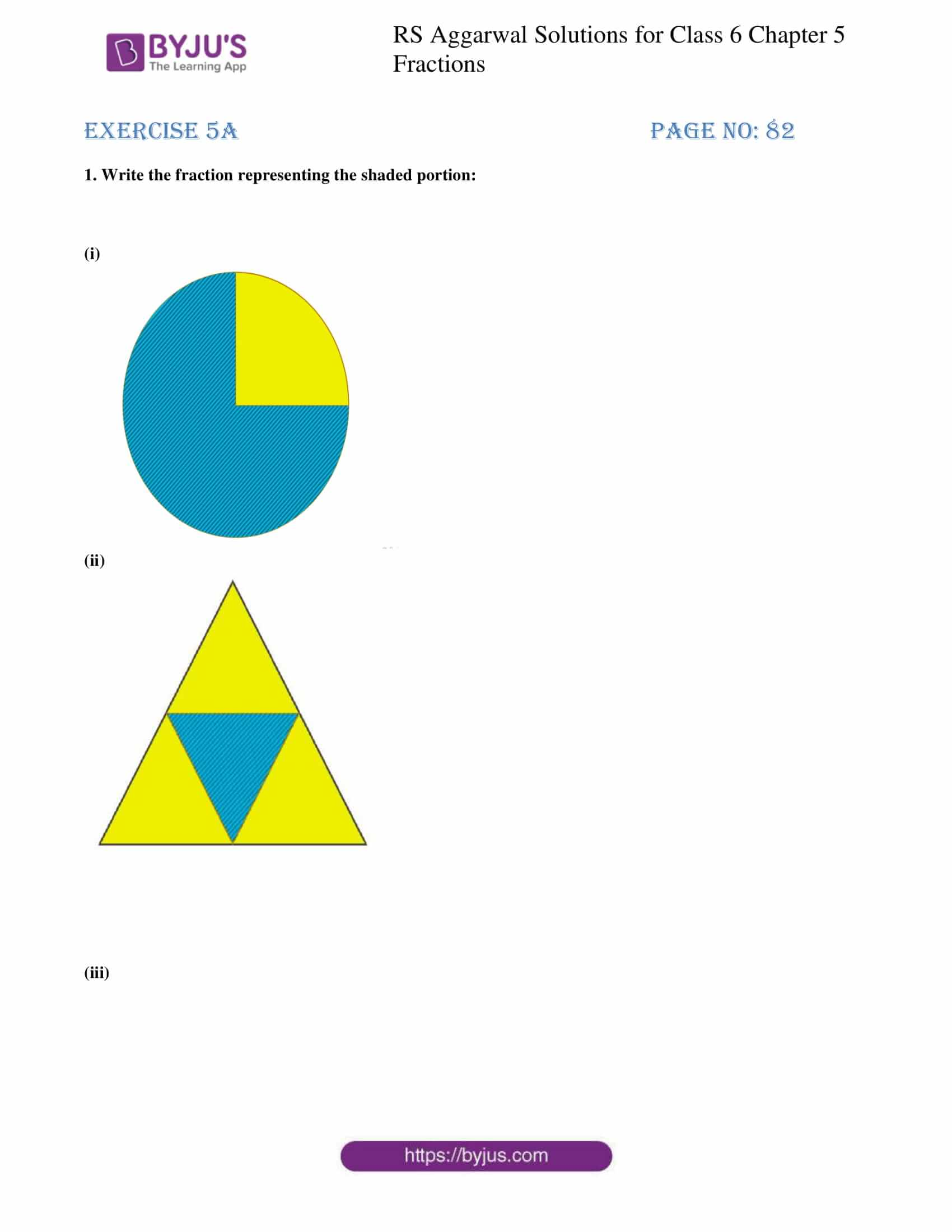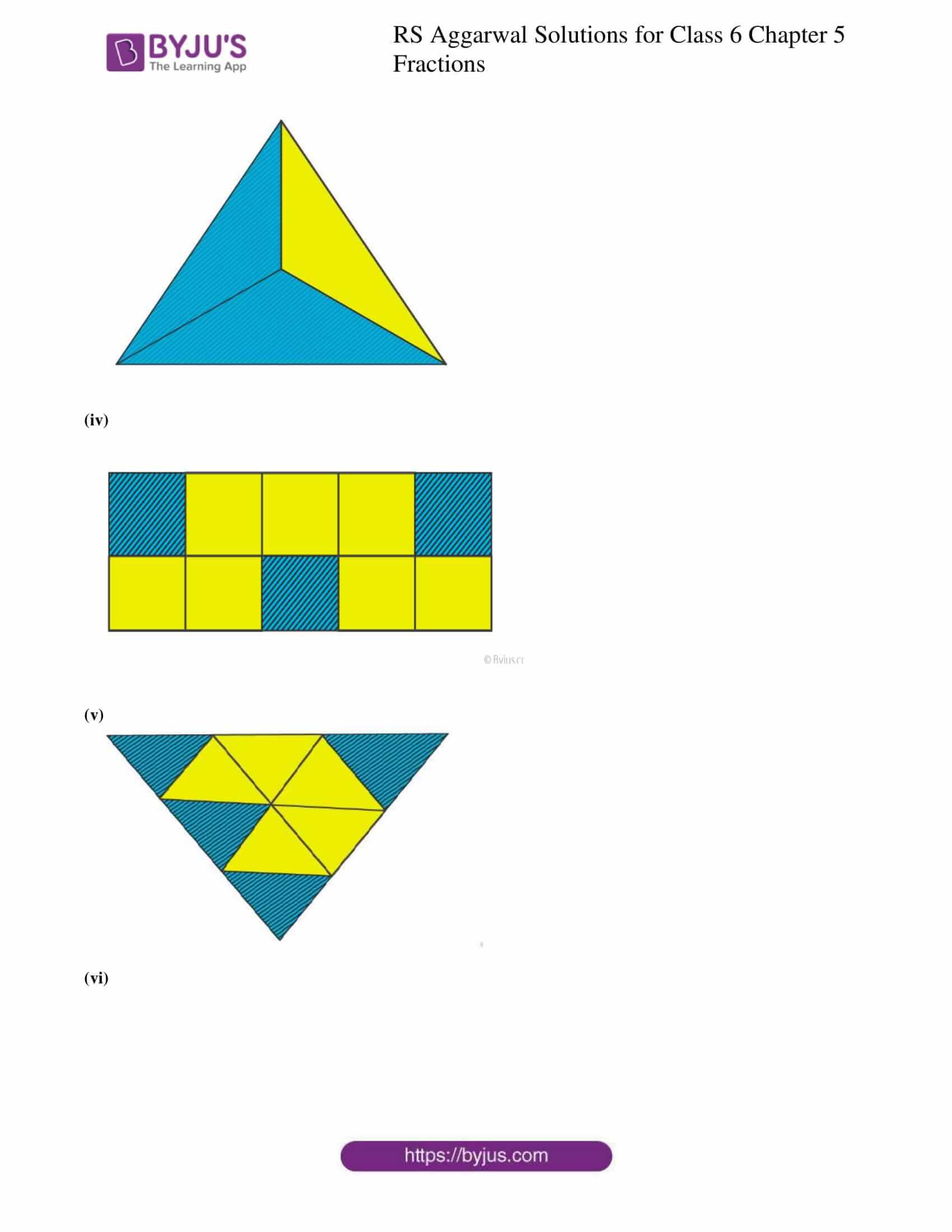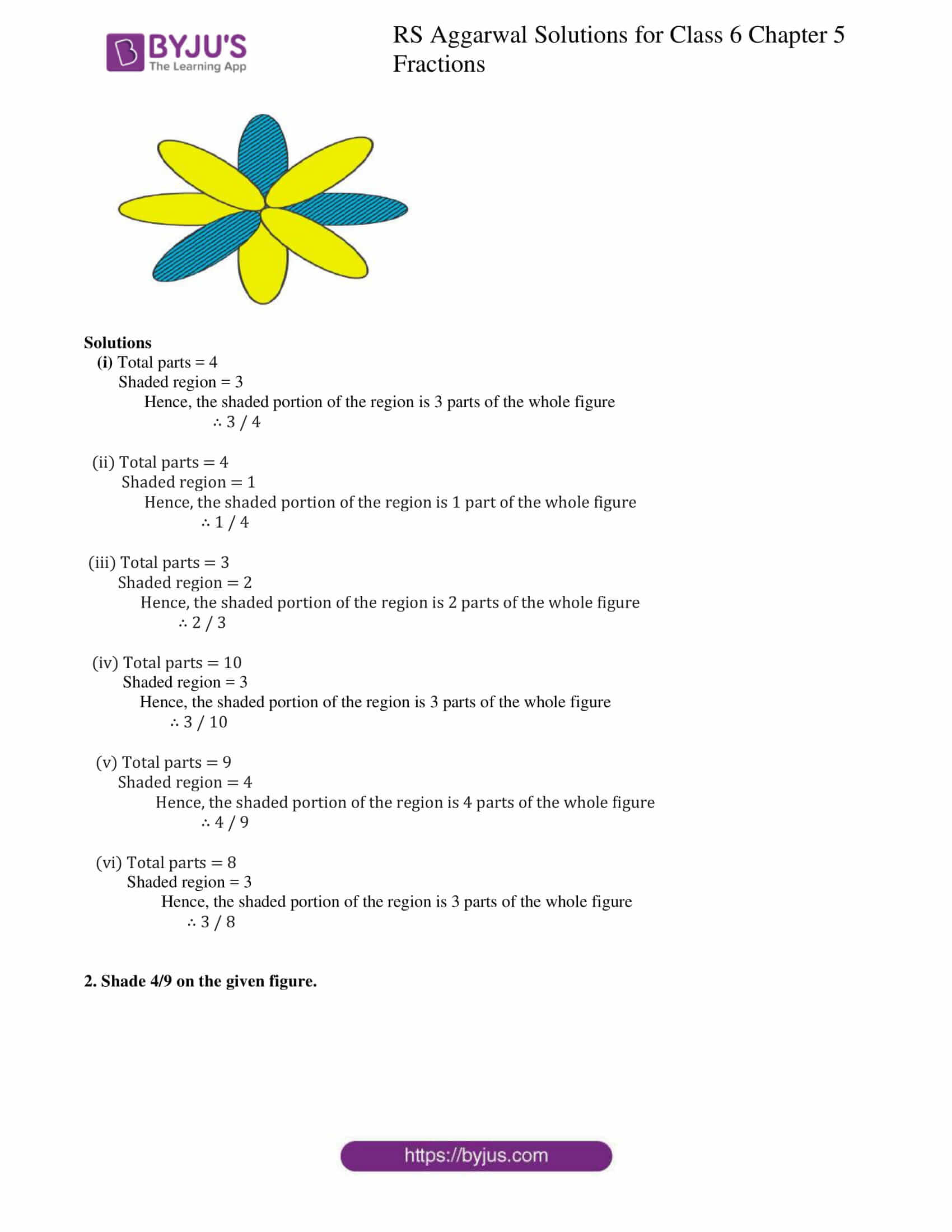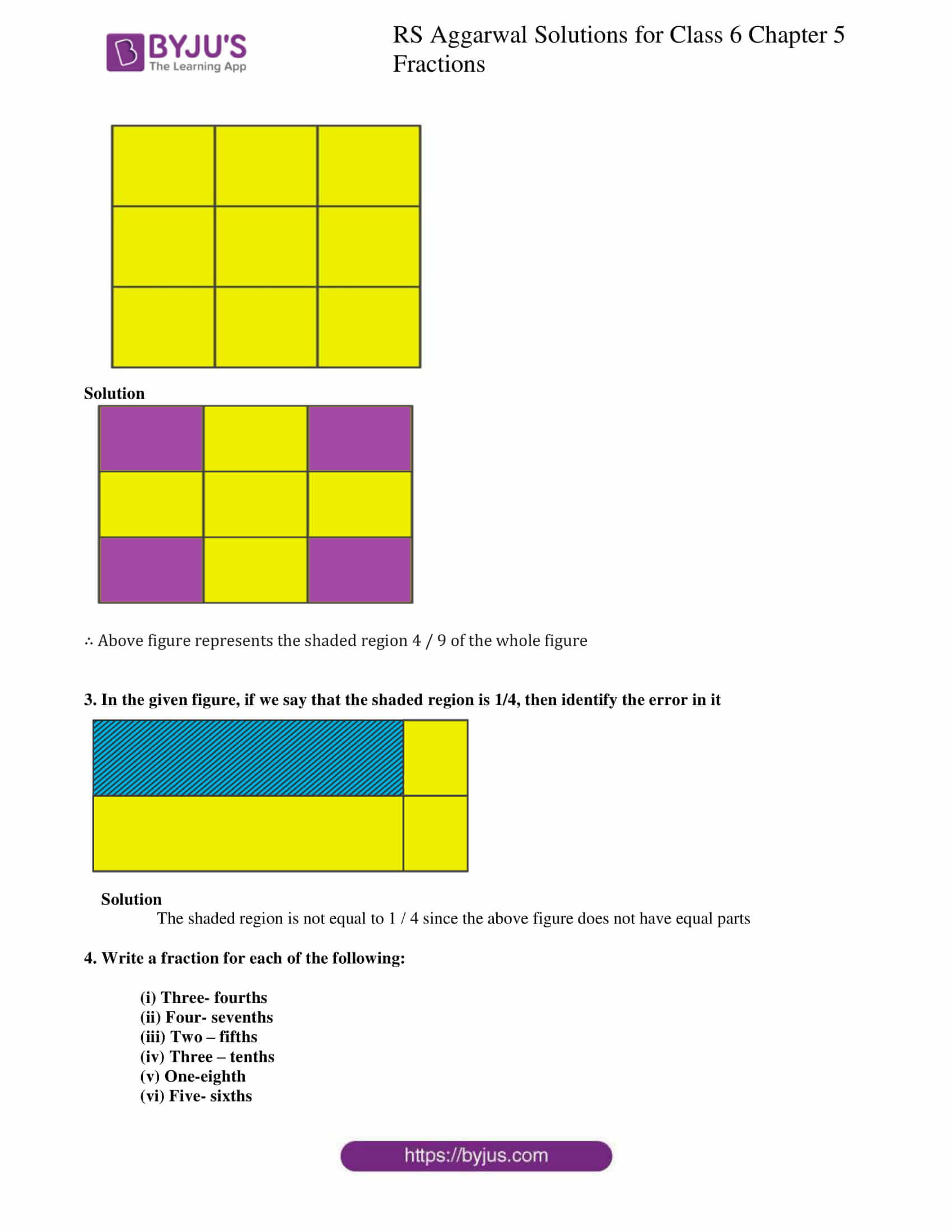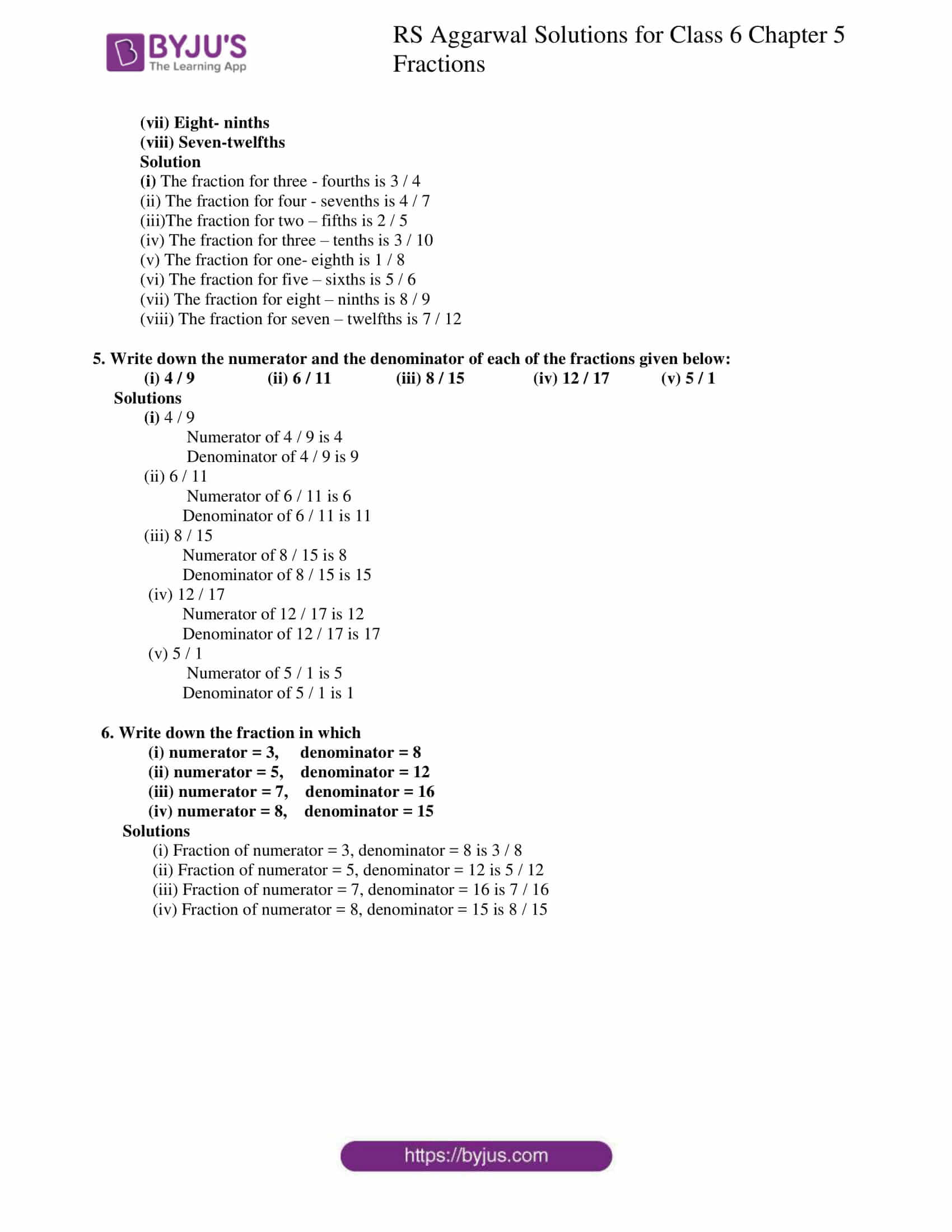### Access answers to Maths RS Aggarwal Solutions for Class 6 Chapter 5 Fractions Exercise 5A

1. Write the fraction representing the shaded portion:

(i)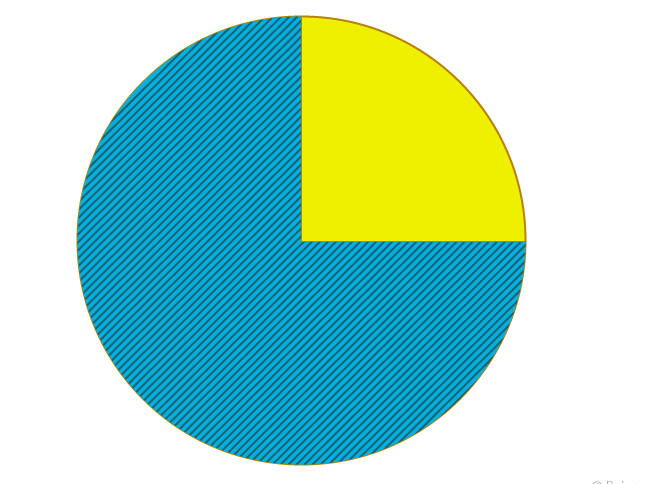(ii)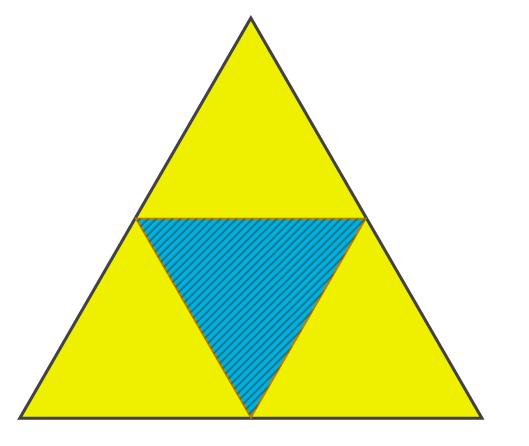(iii)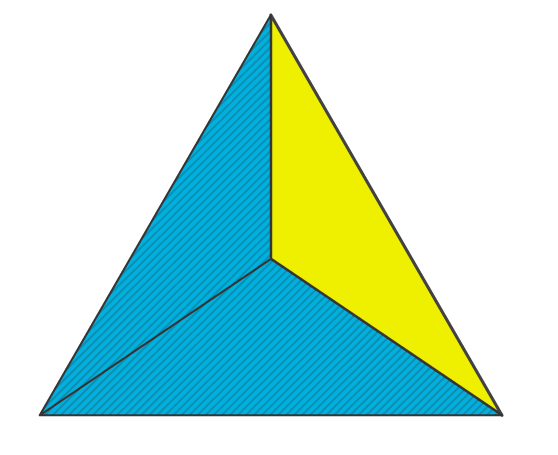(iv)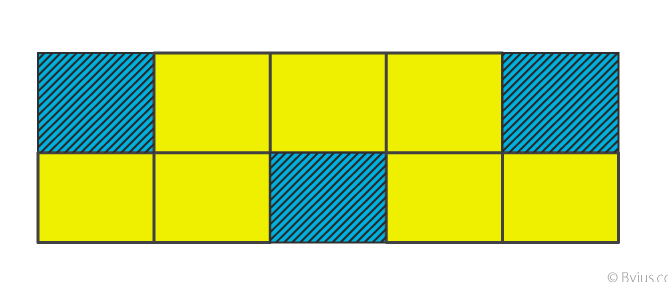(v)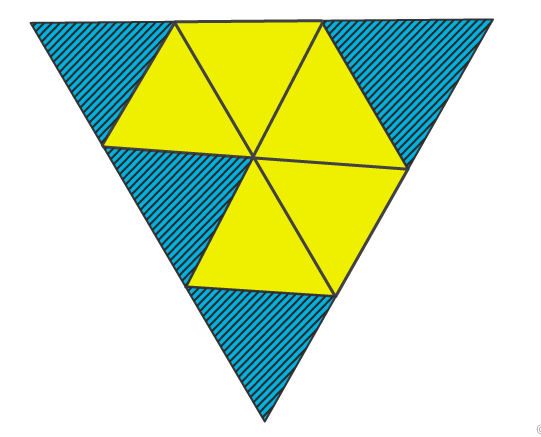(vi)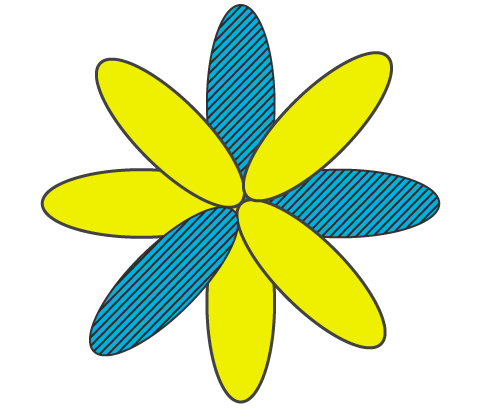Solutions

(i) Total parts = 4

Hence, the shaded portion of the region is 3 parts of the whole figure

∴ 3 / 4

(ii) Total parts = 4

Hence, the shaded portion of the region is 1 part of the whole figure

∴ 1 / 4

(iii) Total parts = 3

Hence, the shaded portion of the region is 2 parts of the whole figure

∴ 2 / 3

(iv) Total parts = 10

Hence, the shaded portion of the region is 3 parts of the whole figure

∴ 3 / 10

(v) Total parts = 9

Hence, the shaded portion of the region is 4 parts of the whole figure

∴ 4 / 9

(vi) Total parts = 8

Hence, the shaded portion of the region is 3 parts of the whole figure

∴ 3 / 8

2. Shade 4/9 on the given figure.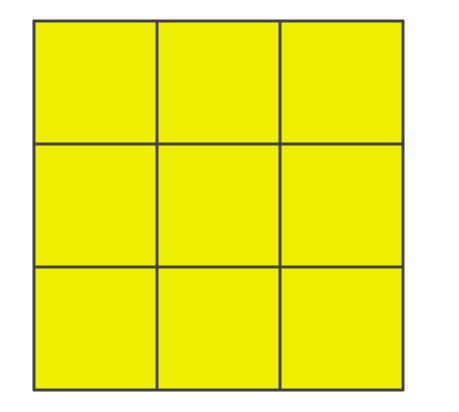Solution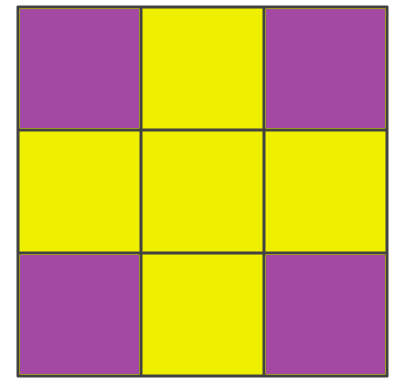∴ Above figure represents the shaded region 4 / 9 of the whole figure

3. In the given figure, if we say that the shaded region is 1/4, then identify the error in it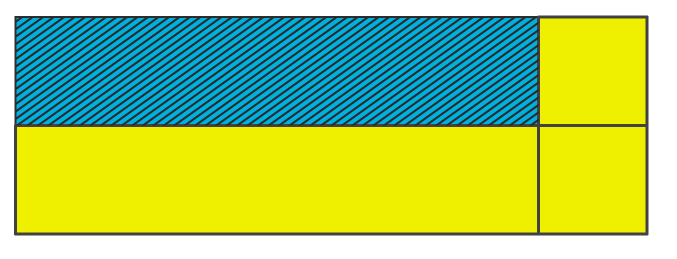Solution

The shaded region is not equal to 1 / 4 since the above figure does not have equal parts

4. Write a fraction for each of the following:

(i) Three- fourths

(ii) Four- sevenths

(iii) Two – fifths

(iv) Three – tenths

(v) One-eighth

(vi) Five- sixths

(vii) Eight- ninths

(viii) Seven-twelfths

Solution

(i) The fraction for three – fourths is 3 / 4

(ii) The fraction for four – sevenths is 4 / 7

(iii)The fraction for two – fifths is 2 / 5

(iv) The fraction for three – tenths is 3 / 10

(v) The fraction for one- eighth is 1 / 8

(vi) The fraction for five – sixths is 5 / 6

(vii) The fraction for eight – ninths is 8 / 9

(viii) The fraction for seven – twelfths is 7 / 12

5. Write down the numerator and the denominator of each of the fractions given below:

(i) 4 / 9 (ii) 6 / 11 (iii) 8 / 15 (iv) 12 / 17 (v) 5 / 1

Solutions

(i) 4 / 9

Numerator of 4 / 9 is 4

Denominator of 4 / 9 is 9

(ii) 6 / 11

Numerator of 6 / 11 is 6

Denominator of 6 / 11 is 11

(iii) 8 / 15

Numerator of 8 / 15 is 8

Denominator of 8 / 15 is 15

(iv) 12 / 17

Numerator of 12 / 17 is 12

Denominator of 12 / 17 is 17

(v) 5 / 1

Numerator of 5 / 1 is 5

Denominator of 5 / 1 is 1

6. Write down the fraction in which

(i) numerator = 3, denominator = 8

(ii) numerator = 5, denominator = 12

(iii) numerator = 7, denominator = 16

(iv) numerator = 8, denominator = 15

Solutions

(i) Fraction of numerator = 3, denominator = 8 is 3 / 8

(ii) Fraction of numerator = 5, denominator = 12 is 5 / 12

(iii) Fraction of numerator = 7, denominator = 16 is 7 / 16

(iv) Fraction of numerator = 8, denominator = 15 is 8 / 15